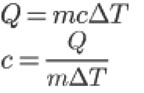SEARCH HOMEMath Central Quandaries & QueriesQuestion from erin, a student: Hi- 2 kg metal requires 1.00 X 104 J of heat to raise its temperature from 20 degrees C to 40 degrees C. What is the specific heat capacity of the metal?Hi Erin,

Find the specific heat is fairly simple if you know the formula:Where Q is the heat m is the mass c is specific heat of the substance ΔT is change in temperature (ΔT = Final temperature - Initial Temperature)

If you rearrange the first formula and solve for c, you can just plug in your numbers and find specific heat.

Hope this helps,

JaniceMath Central is supported by the University of Regina and The Pacific Institute for the Mathematical Sciences.